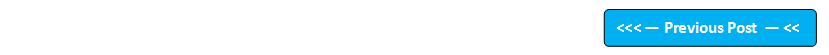## Blog Pages

### 222. Catching arithmetic exceptions using ArithmeticException ClassPre-requisite -

ArithmeticException Class can handle the arithmetic exceptions thrown by the code directly. No need of upcasting in this case, as arithmetic exception (object) can be assigned directly to the ArithmeticException Class object. And also -
• ArithmeticException is the sub-class of Exception Class
• ArithmeticException falls into the Run Time Exceptions category of Exception Class
• ArithmeticException class can directly handle the arithmetic exceptions thrown by the code.

Example -

Lets say we got an Arithmetic exception in the code as shown below. When we handle this exception using the try{ } catch{ } blocks, try{ } will throw the exception (i.e. object) of the 'ArithmeticException' class and catch block catches the exception into the ArithmeticException Class object directly (i.e. without any casting needed) -

class ClassOne
{
try
{
int i = 5/0;  //Throws Arithmetic Exception
}

catch(ArithmeticException object1)
{
System.out.println("Exception Details - "+object1);
}

}

Lets implement this on Eclipse IDE -

1. Launch Eclipse IDE, in the existing Java Project 'Project 46', create a Class 'ArithmeticExceptionDemo' with main( ) method as shown below -

2. Inside the main( ) method, intentionally write the statement int i = 5/0; which throws Arithmetic Exception as shown below -

3. Now handle the exception using the try{ } catch{ } blocks by first placing the statement which throws the arithmetic exception in try{ } block as shown below -

4. Now write the catch{ } block which directly catches the Arithmetic exception thrown by the try{} block into the ArithmeticException Class object as shown below -

5. Now lets print the occurred arithmetic exception using the ArithmeticException Class object as shown below -

6. Run the Java Class file 'ArithmeticExceptionDemo.java' and observe that the arithmetic exception details are displayed in the output using the object of ArithmeticException Class in the above code as shown below -

Hence we can catch the arithmetic exceptions directly using the ArithmeticException Class without casting the thrown object.